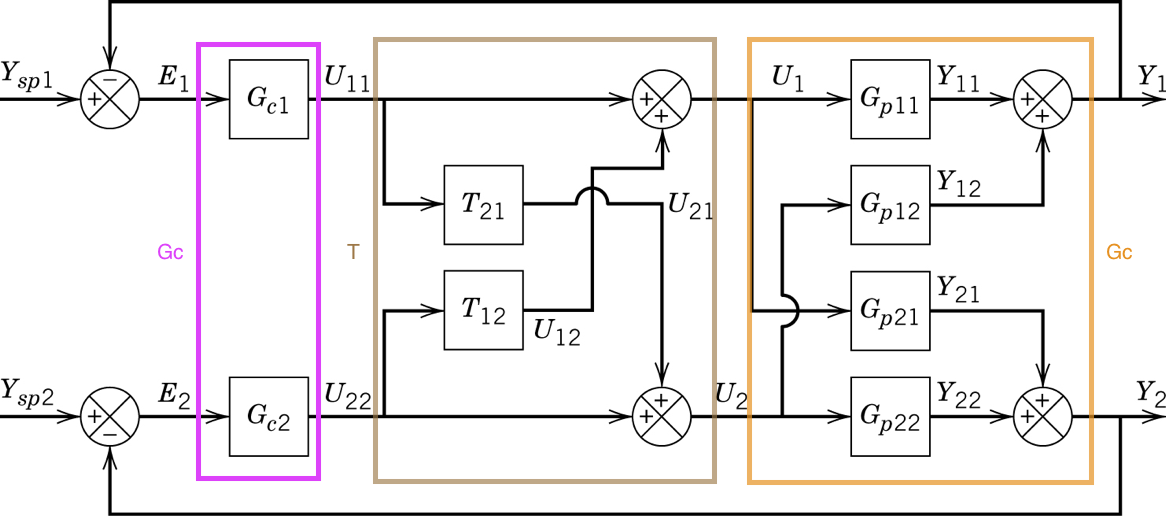# 86. Decoupling¶

Given a general multivariable system with transfer function matrix $$G_p$$, a decoupler attempts to combine with the system to form a diagonal whole.:

import sympy
sympy.init_printing()

:

G_p11, G_p12, G_p21, G_p22 = sympy.symbols('G_p11, G_p12, G_p21, G_p22')
G_p = sympy.Matrix([[G_p11, G_p12],[G_p21, G_p22]])
G_p

:

$$\left[\begin{matrix}G_{p11} & G_{p12}\\G_{p21} & G_{p22}\end{matrix}\right]$$

## 86.1. 1. Inverse-based¶

Wouldn’t it be nice if the system didn’t have interaction? In other words, we could choose $$T$$ such that we have this system with the same diagonal elements as the original system but zeros in the off diagonals.

:

G_s = sympy.Matrix([[G_p11, 0],[0, G_p22]])
G_s

:

$$\left[\begin{matrix}G_{p11} & 0\\0 & G_{p22}\end{matrix}\right]$$

Recalling that the combination of $$T$$ and $$G_p$$ in series is $$G_p T$$, we can solve for the decoupler directly

$G_P T = G_s \therefore T = G_P^{-1} G_s$
:

T = G_p.inv()*G_s
T

:

$$\left[\begin{matrix}\frac{G_{p11} G_{p22}}{G_{p11} G_{p22} - G_{p12} G_{p21}} & - \frac{G_{p12} G_{p22}}{G_{p11} G_{p22} - G_{p12} G_{p21}}\\- \frac{G_{p11} G_{p21}}{G_{p11} G_{p22} - G_{p12} G_{p21}} & \frac{G_{p11} G_{p22}}{G_{p11} G_{p22} - G_{p12} G_{p21}}\end{matrix}\right]$$

Let’s see if that worked:

:

G_pT = G_p*T
sympy.simplify(G_pT)

:

$$\left[\begin{matrix}G_{p11} & 0\\0 & G_{p22}\end{matrix}\right]$$

Pros:

• Controller design can be based on open loop model
• Apparent dynamics (what the controller sees) are simple

Cons:

• T is often not physically realisable
• T is complicated

## 86.2. 2. Zero off-diagonals¶

A more common strategy is to solve directly for the off-diagonal elements of set equal to zero.

So we just want

$\begin{split}G_P T = \begin{bmatrix}d_1&0\\0&d_2\end{bmatrix}\end{split}$

Note the difference between the first method and this one - here we are not specifying the diagonal at all, we just want the off-diagonals to be zero.

:

T21, T12 = sympy.symbols('T21, T12')
T = sympy.Matrix([[1, T12],
[T21, 1]])

wantdiagonal = G_p*T

sol = sympy.solve([wantdiagonal[0,1], wantdiagonal[1, 0]], [T21, T12])

:

T.subs(sol)

:

$$\left[\begin{matrix}1 & - \frac{G_{p12}}{G_{p11}}\\- \frac{G_{p21}}{G_{p22}} & 1\end{matrix}\right]$$

So this is the classic/traditional decoupler shown in the diagram (with unit passthrough on the diagonals). This changes the transfer function the controller “sees” to

:

G_p*T.subs(sol)

:

$$\left[\begin{matrix}G_{p11} - \frac{G_{p12} G_{p21}}{G_{p22}} & 0\\0 & G_{p22} - \frac{G_{p12} G_{p21}}{G_{p11}}\end{matrix}\right]$$

Pros:

• Relatively simple design process
• Less complicated decoupler than the inverse-based method

Cons:

• Apparent plant may be higher order than the actual plant
• Still requires an inverse, may not be physically realisable (but more likely than method 1)

The adjugate (previously calld the adjoint) of a matrix will also diagonalise a system

:

T = G_p.adjugate()
T

:

$$\left[\begin{matrix}G_{p22} & - G_{p12}\\- G_{p21} & G_{p11}\end{matrix}\right]$$
:

G_p*T

:

$$\left[\begin{matrix}G_{p11} G_{p22} - G_{p12} G_{p21} & 0\\0 & G_{p11} G_{p22} - G_{p12} G_{p21}\end{matrix}\right]$$

Pros:

• Decoupler guaranteed to be physically realisable because it only requires “forward” models of the system.

Cons:

• Apparent plant now much higher order (look at the products in the $$G_pT$$ expression)
[ ]: